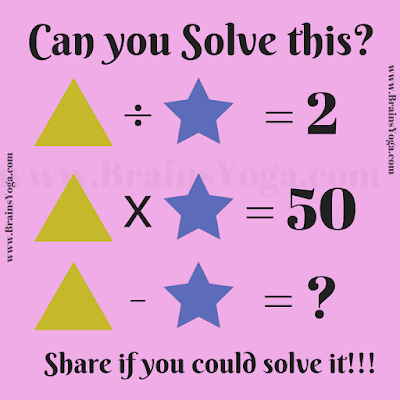Here is simple Mathematical equation for kids. In this Maths equation you challenge is to find the value of given variable Triangle and Star and then solve the last equation. Can you solve it as quickly as possible?Can you solve this Maths Equation?